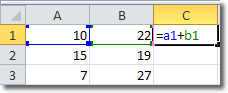Planet For Application Life Development Presents MY IT WorldExplore and uptodate your technology skills...

Excel - Absolute Reference

By default, cell references in Excel are relative. For example, if you have the following formula in cell C1:and you copy the formula to cell C2, you will get =A2+B2. This is because the cell references are relative. The contents of cell C1 are calculated to be the sum of the the cell that is 2 cells to the left and the cell that is 1 cell to the left. This easily transposes to cell C2.

To demonstrate, I copied cell C1 to cell C2 and got this:You can see in the formula bar that the original formula of =A1+B2 has been transformed to =A2+B2 when copied to cell C2.

But what if we want to always use A1+B1 in all cells we copy that formula to? This is where absolute cell references come in.

How To Construct An Absolute Cell Reference

In Excel, we create an absolute cell reference by using a “\$” before each letter and number. The formula for C1 then becomes

=\$A\$1+\$B\$2

Copying that formula to C2 retains those absolute references and we find the that C2 also uses =\$A\$1+\$B\$2.

You can also mix ansolute with relative references like this: A\$1. Here the row is fixed at one, but the column remains relative.